# NCERT Solutions class-11 Chemistry The s-Block Elements Part-2## myCBSEguide App

Complete Guide for CBSE Students

NCERT Solutions, NCERT Exemplars, Revison Notes, Free Videos, CBSE Papers, MCQ Tests & more.

16. Starting with sodium chloride how would you proceed to prepare (i) sodium metal (ii) sodium hydroxide (iii) sodium peroxide (iv) sodium carbonate?

17. What happens when (i) magnesium is burnt in air (ii) quick lime is heated with silica (iii) chlorine reacts with slaked lime (iv) calcium nitrate is heated ?

18. Describe two important uses of each of the following: (i) caustic soda (ii) sodium carbonate (iii) quicklime.

19. Draw the structure of (i)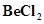(vapour) (ii)(solid).

20. The hydroxides and carbonates of sodium and potassium are easily soluble in water while the corresponding salts of magnesium and calcium are sparingly soluble in water. Explain.

21. Describe the importance of the following: (i) limestone (ii) cement (iii) plaster of paris.

Importance of plaster of Paris

22. Why are lithium salts commonly hydrated and those of the other alkali metal ions usually anhydrous?

23. Why is LiF almost insoluble in water whereas LiCl soluble not only in water but also in acetone?

24. Explain the significance of sodium, potassium, magnesium and calcium inbiological fluids.

25. What happens when

(i) sodium metal is dropped in water?

(ii) sodium metal is heated in free supply of air?

(iii) sodium peroxide dissolves in water?

26. Comment on each of the following observations:

(a) The mobilities of the alkali metal ions in aqueous solution are Li+< Na+< K+ < Rb+ < Cs+

(b) Lithium is the only alkali metal to form a nitride directly.

(c)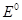for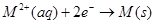(where M = Ca, Sr or Ba) is nearly constant.

27. State as to why

(a) a solution of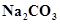is alkaline?

(b) alkali metals are prepared by electrolysis of their fused chlorides?

(c) sodium is found to be more useful than potassium?

28. Write balanced equations for reactions between

(a)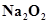and water

(b)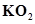and water

(c)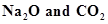29. How would you explain the following observations?

(i) BeO is almost insoluble but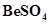in soluble in water,

(ii) BaO is soluble but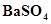is insoluble in water,

(iii) LiI is more soluble than KI in ethanol.
30. Which of the alkali metal is having least melting point?

(a) Na

(b) K

(c) Rb

(d) Cs

31. Which one of the following alkali metals gives hydrated salts?

(a) Li

(b) Na

(c) K

(d) Cs

32. Which one of the alkaline earth metal carbonates is thermally the most stable?

(a)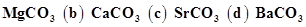## Test Generator

Create Papers with your Name & Logo